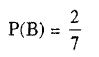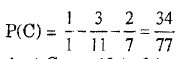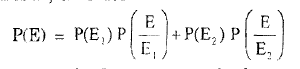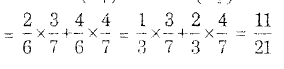# Engineering Maths - Probability

>>>>>>>>Probability

• A

less than P(A) + P(B) - 1• B

greater than P(A) + P(B)• C

equal to P(A) + P(B) - P(A ∪ B)• D

equal to P(A) + P(B) + P(A ∪ B)• A

P(M) + P(N) - 2P(M ∩ N)• B

P(M) + P(N) - P(M ∩ N)• C

P(M') + P(N') - 2P(M' ∩ N')• D

P(M ∩ N') + P(M' ∩ N)• A

A ∪ B and A ∩ B'• B

A ∪ B and A' ∩ B• C

A ∪ B and A' ∩ B'• D

A ∪ B and A ∩ B• A

43 to 34• B

32 to 23• C

34 to 13• D

none of these• Option : A
• Explanation : Since odds are 8 to 3 against Aand                                   P(A) + P(B) + P(C) = 1Hence odds against C are 43 to 34.

• A

11/21• B

21/11• C

12/21• D

21/12• Option : A
• Explanation : Let E1 be the event that a ball is drawn from
first bag, E2 the event that a ball is drawn
from second bag and E the event a black ball
is chosen, thereforeRelated Quiz.
Probability